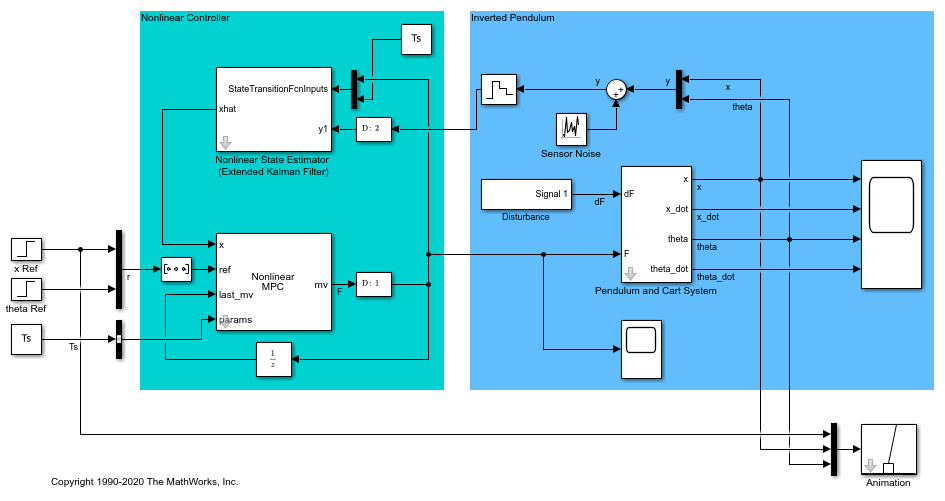createParameterBus

Create Simulink bus object and configure Bus Creator block for passing model parameters to Nonlinear MPC Controller block

Description

example

createParameterBus(nlmpcobj,nlmpcblk,busName,parameters) creates a Simulink.Bus object, busName, in the MATLAB® workspace for passing model parameters to a Nonlinear MPC Controller block, nlmpcblk. createParameterBus requires you to connect a Bus Creator block to the Nonlinear MPC Controller block in advance so that it can configure the Bus Creator block to use the bus object.

Examples

collapse all

Create a nonlinear MPC controller with four states, two outputs, and one input.

nlobj = nlmpc(4,2,1);
In standard cost function, zero weights are applied by default to one or more OVs because there are fewer MVs than OVs.

Specify the sample time and horizons of the controller.

Ts = 0.1;
nlobj.Ts = Ts;
nlobj.PredictionHorizon = 10;
nlobj.ControlHorizon = 5;

Specify the state function for the controller, which is in the file pendulumDT0.m. This discrete-time model integrates the continuous-time model defined in pendulumCT0.m using a multistep forward Euler method.

nlobj.Model.StateFcn = "pendulumDT0";
nlobj.Model.IsContinuousTime = false;

The prediction model uses an optional parameter, Ts, to represent the sample time. Specify the number of parameters.

nlobj.Model.NumberOfParameters = 1;

Specify the output function of the model, passing the sample-time parameter as an input argument.

nlobj.Model.OutputFcn = @(x,u,Ts) [x(1); x(3)];

Define standard constraints for the controller.

nlobj.Weights.OutputVariables = [3 3];
nlobj.Weights.ManipulatedVariablesRate = 0.1;
nlobj.OV(1).Min = -10;
nlobj.OV(1).Max = 10;
nlobj.MV.Min = -100;
nlobj.MV.Max = 100;

mdl = 'mpc_pendcartNMPC';
open_system(mdl)In this model, the Nonlinear MPC Controller block is configured to use the controller nlobj.

To use the optional parameter in the prediction model, the model has a Simulink Bus block connected to the params input port of the Nonlinear MPC Controller block. To configure this bus block to use the Ts parameter, create a Bus object in the MATLAB® workspace, and configure the Bus Creator block to use this object. Name the Bus object 'myBusObject'.

createParameterBus(nlobj,[mdl '/Nonlinear MPC Controller'],'myBusObject',{Ts});

bdclose(mdl)
A Simulink Bus object "myBusObject" created in the MATLAB Workspace, and Bus Creator block "mpc_pendcartNMPC/Nonlinear MPC Controller" is configured to use it.

Input Arguments

collapse all

Nonlinear MPC controller, specified as an nlmpc object.

Block path of Nonlinear MPC Controller block, specified as a string or character vector.

Name of Simulink bus object to be created in the MATLAB workspace and set in the Bus Creator block, specified as a string or character vector.

The corresponding Bus Creator block must already be connected to the params input port of the Nonlinear MPC Controller block specified by nlmpcblk. Also, the Bus Creator block must have the correct number of input ports, and these ports must already be properly connected.

Nominal prediction model parameter values, specified as a cell array of length Np, where Np is equal to nlmpcobj.Model.NumberOfParameters. The order of the parameters must match the order specified in the model functions, and each parameter must be a numeric parameter with the correct dimensions.